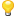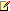Chinese Simplified (简体中文)

# 支持的 OCR 和文档对比语言

ABBYY FineReader PDF 支持 201 种 OCR 语言：支持的语言的可能因产品版本的不同而不同。

• 阿布哈兹语
• 阿瓦尔语
• 阿古尔语
• 阿迪格语
• 阿塞拜疆语（西里尔）, 阿塞拜疆语（拉丁）**
• 艾玛拉语
• 阿尔巴尼亚语
• 阿尔泰语
• 英语音标
• 英语*, **
• 阿拉伯语（沙特阿拉伯）
• 亚美尼亚语 （东部、西部、格拉巴）*
• 南非荷兰语
• 那瓦特语
• 巴斯克语
• 巴什基尔语*, **
• 白俄罗斯语
• 本巴语
• 布莱克福特语
• 保加利亚语*, **
• 布列塔尼语
• 布哥图语
• 布里亚特语
• 威尔士语
• 匈牙利语*, **
• 沃洛夫语
• 越南语*, **
• 夏威夷语
• 加告兹语
• 加利西亚语
• 干达语
• 希腊语*, **
• 瓜拉尼语
• 卡瓦语
• 苏格兰盖尔语
• 达科他语
• 达尔格瓦语
• 丹麦语*, **
• 侗族语
• 东干语
• 祖鲁语
• 希伯来语*
• 依地语
• 印古什语
• 印度尼西亚语*, **
• 爱尔兰语
• 冰岛语
• 西班牙语*, **
• 意大利语*, **
• 卡巴尔达语
• 哈萨克语
• 卡尔梅克语
• 卡拉卡尔帕克人
• 恰伊-巴尔卡尔语
• 加泰隆语*, **
• 卡舒比语
• 凯楚阿语（玻利维亚）
• 吉库犹语
• 吉尔吉斯语
• 简体中文**, 繁体中文
• 刚果语
• 朝鲜语, 韩国语（韩文字母）
• 科西嘉语
• 科尔雅克语
• 科萨语
• 克佩勒语
• 克罗语
• 克里米亚鞑靼语
• 库梅克语
• 库尔德语
• 拉克语
• 拉丁语*
• 拉脱维亚语*, **
• 莱兹吉语
• 立陶宛语*, **
• 卢巴语
• 索布语
• 玛雅语
• 马其顿语
• 马达加斯加语
• 马来语（马来西亚）
• 马林凯语
• 马耳他语
• 曼西语
• 毛利语
• 马里语
• 米南卡堡语
• 莫霍克语
• 罗马尼亚语 （摩尔瓦多）
• 蒙古语
• 摩尔多瓦语
• 苗语
• 德语（卢森堡）
• 德语**, 德语（新的拼写规则）*, **
• 勒勒茨语
• 尼夫赫语
• 荷兰语**, 荷兰语（比利时）*, **
• 诺盖语
• 挪威语（尼诺斯克）**, 挪威语（博克马尔）*, **
• 尼昂加语
• 奥杰布瓦语
• 奥塞梯语
• 帕皮阿门托语
• 波兰语*, **
• 葡萄牙语**, 葡萄牙语（巴西）*, **
• 奥克语
• 利托-罗曼诸语
• 卢旺达语
• 罗马尼亚语*, **
• 隆迪语
• 俄语*, **
• 俄语（旧式拼写）
• 俄语带重音标记
• 萨米语（拉皮什）
• 萨摩亚语
• 萨巴特克语
• 斯瓦齐语
• 宿务语
• 塞尔库普语
• 塞尔维亚语（西里尔）, 塞尔维亚语（拉丁）
• 斯洛伐克语*, **
• 斯洛文尼亚语*, **
• 索马里语
• 斯瓦希利语
• 巽他語
• 塔巴萨兰语
• 塔加洛语
• 塔吉克语
• 塔希提语
• 泰国语*
• 鞑靼语*, **
• 托克皮辛语
• 汤加语
• 茨瓦纳语
• 图瓦语
• 土耳其语*, **
• 土库曼语（西里尔）, 土库曼语（拉丁）
• 乌德穆尔特语
• 维吾尔语（西里尔）, 维吾尔语（拉丁）
• 乌兹别克语（西里尔）, 乌兹别克语（拉丁）
• 乌克兰语*, **
• 法罗语
• 斐济语
• 芬兰语*, **
• 法语*, **
• 弗里西亚语
• 弗留利语
• 哈卡斯语
• 哈尼语
• 汉特语
• 豪萨语
• 克罗地亚语*, **
• 景颇语
• 吉普赛语
• 查莫罗语
• 车臣语
• 捷克语*, **
• 楚瓦什语
• 楚克其语
• 瑞典语*, **
• 修纳语
• 鄂温克语
• 鄂温语
• 爱斯基摩语（西里尔）, 爱斯基摩语（拉丁）
• 爱沙尼亚语*, **
• 科萨语
• 雅库特语
• 日语

• 旧式英语*
• 旧式法语*
• 旧式德语*
• 旧式意大利语*
• 旧式西班牙语*
• 拉脱维亚哥特式

• 伊多语
• 国际语
• 西方语
• 世界语

• Basic
• C/C++
• COBOL
• Fortran
• Java
• Pascal
• 简单数学公式
• 数字有关显示特定语言字符所需字体的信息，请参阅 以支持的语言正确显示文本所需的字体